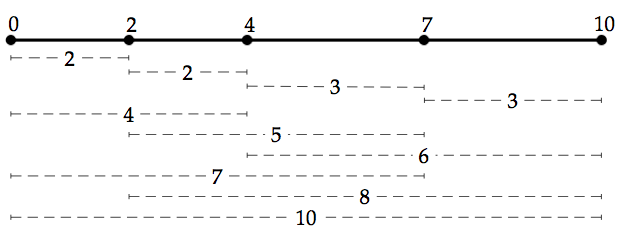# Creating a Restriction Map solved by 297

Jan. 11, 2013, 6:26 p.m. by Rosalind Team

Topics: Set Theory

## Genetic Fingerprinting

Recall that a restriction enzyme cuts the endpoints of a specific interval of DNA, which must form a reverse palindrome that typically has length 4 or 6. The interval of DNA cleaved by a given restriction enzyme is called its recognition sequence.

A single human chromosome is so long that a given recognition sequence will occur frequently throughout the chromosome (recall from “Expected Number of Restriction Sites” that a recognition sequence would be expected to occur several times even in a short chromosome). Nevertheless, the small-scale mutations that create diversity in the human genome (chiefly SNPs) will cause each human to have a different collection of recognition sequences for a given restriction enzyme.

Genetic fingerprinting is the term applied to the general process of forming a limited picture of a person's genetic makeup (which was traditionally cheaper than sequencing). The earliest application of genetic fingerprinting inexpensive enough to be widely used in common applications, like forensics and paternity tests, relied on a process called restriction digest. In this technique, a sample of DNA is replicated artificially, then treated with a given restriction enzyme; when the enzyme cuts the DNA at restriction sites, it forms a number of fragments. A second process called gel electrophoresis then separates these fragments along a membrane based on their size, with larger pieces tending toward one end and smaller pieces tending toward the other. When the membrane is stained or viewed with an X-ray machine, the fragments create a distinct banding pattern, which typically differs for any two individuals.

These intervals can be thought of simply as the collection of distances between restriction sites in the genome. Before the rapid advances of genome sequencing, biologists wanted to know if they could use only these distances to reconstruct the actual locations of restriction sites in the genome, forming a restriction map. Restriction maps were desired in the years before the advent of sequencing, when any information at all about genomic makeup was highly coveted. The application of forming a restriction map from cleaved restriction fragments motivates the following problem.

## ProblemFigure 1. In the simplified figure above, we know that the dashed segments came from a chromosome; we desire a collection of numbers whose differences match the lengths of the dotted lines, which will correspond to the locations of restriction sites on the unknown chromosome. Taken from Jones & Pevzner, An Introduction to Bioinformatics Algorithms.

For a set $X$ containing numbers, the difference multiset of $X$ is the multiset $\Delta X$ defined as the collection of all positive differences between elements of $X$. As a quick example, if $X =\{2, 4, 7\}$, then we will have that $\Delta X = \{2, 3, 5\}$.

If $X$ contains $n$ elements, then $\Delta X$ will contain one element for each pair of elements from $X$, so that $\Delta X$ contains $\binom{n}{2}$ elements (see combination statistic). You may note the similarity between the difference multiset and the Minkowski difference $X \ominus X$, which contains the elements of $\Delta X$ and their negatives. For the above set $X$, $X \ominus X$ is $\{-5, -3, -2, 2, 3, 5\}$.

In practical terms, we can easily obtain a multiset $L$ corresponding to the distances between restriction sites on a chromosome. If we can find a set $X$ whose difference multiset $\Delta X$ is equal to $L$, then $X$ will represent possible locations of these restriction sites. For an example, consult Figure 1.

Given: A multiset $L$ containing $\binom{n}{2}$ positive integers for some positive integer $n$.

Return: A set $X$ containing $n$ nonnegative integers such that $\Delta X = L$.

## Sample Dataset

2 2 3 3 4 5 6 7 8 10


## Sample Output

0 2 4 7 10